# Change Nickname

Current Nickname:
Search
v2.x
v4.0

# PageRank

Basic

## Overview

PageRank algorithm is an iterative algorithm that passes the scores of nodes along the direction of the directed edges in directed graph until the score distribution of the whole graph converges, the underlying assumption is that "more important web pages are likely to receive more or more important backlinks from other web pages". This algorithm was proposed from 1997 to 1998 by Google co-founders Larry Page and Sergey Brin with the purpose to calculate the popularity and importance of web pages, so as to provide a basis for ranking search results. With the development of technology and the emergence of enormous correlation data, the usage of PageRank has been drived into many other fields.

Related materials of the algorithm are as below:

## Basic Concept

The 1-step neighbor of a node that is connected with an outbound edge of that node is called the forward link of that node; on the contrary, it's called the backlink of that node if it's connected with an inbound edge of the node. When using node to represent web page, forward links of node are the web pages that are referenced by that web page, and backlinks represent the web pages that reference that web page.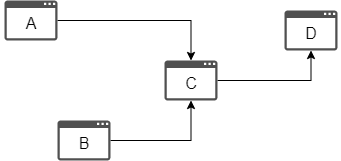Graph: A and B are backlinks of C, D is forward link of C

### PageRank

In the PageRank algorithm, all nodes are assigned an initial score; the score is evenly divided among all outbound edges of the node and passed to each forward link of that node; in the meantime, the node receives score from its various backlinks, and the sum of these received scores is the node's score in this round of transfer: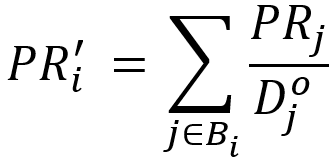In the formula above, node `j` is any backlink of node `i`, `D(j)` is the outbound degree of `j`. For example: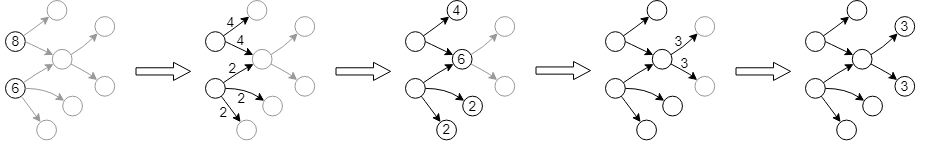When the above scores become steady after multiple rounds of transfer, it can be used to represent the popularity and importance of the web page: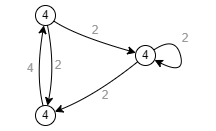### ArticleRank

ArticleRank is an extended application of the PageRank algorithm. The reference of publications is very similar to the general web page reference, but there are also differences. Since a publication cannot reference itself, two publications cannot reference each other, and the references in a published publication do not change, a reference graph of publications has the following features: node does not have self-loop edge, a node cannot be both the forward link and the backlink of another node, and the forward links (outbound degree) of node will not change.

In comparison with PageRank, Ultipa's ArticleRank divides the score of node equally, not by the outbound degree (number of edges in the outbound direction) of that node, but "the sum of the outbound degree of that node and the average outbound degree of nodes in the whole graph" (which also differs from the original ArticleRank). Intuitively, this change will greatly weaken the score transfer capability of nodes with the outbound degree that is much lower than the average outbound degree of the whole graph.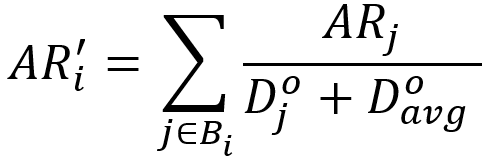In the formula above, `D(avg)` is the average outbound degree of the whole graph. Let's say `D(avg)` is 2, and using this method to re-calculate the previous score transfer process: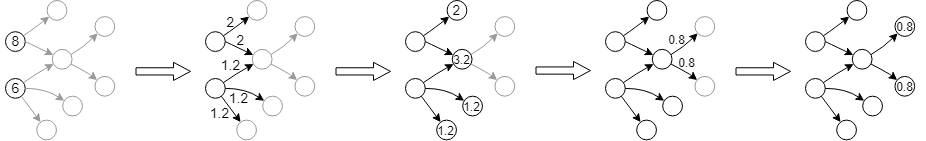There is no cycle in an ideal publication reference graph, thus the total score of the whole graph would decrease as the number of transfer rounds increases, and the score of ArticleRank will never become steady without a damping coefficient.

### Damping Coefficient

Taking PageRank as an example, consider those web pages that are not referenced by any other sites (nodes with inbound degree of 0 and no backlink), such as those lonely web pages, although the score they receive is always 0, they still need to be browsed in the real network; or those web pages that do not reference any other sites (nodes with outbound degree of 0 and no forward link), the score transfer of the whole graph should not become meaningless due to the score loss of these nodes.

To deal with the two cases above as well as the problem that ArticleRank can not converge, damping coefficient - a numeric value between 0 to 1 - is introduced to give each node a floor score while weakening the scores passed from the backlinks (if has) of the node. Take PageRank, for example, when the damping coefficient is `0.7`, each node gets a floor score of `1 - 0.7 = 0.3`, let's say the score a node receives is `8`, it will be weakened to `8 * 0.7 = 5.6` instead, and the sum of the two parts is the score of the node in this round: `0.3 + 5.6 = 5.9`.

The figure below shows a steady state of PageRank calculation with damping coefficient equals to `0.8`, and the initial socre of each node is 1 (please see Algorithm Process - Example below for the result of each iteration):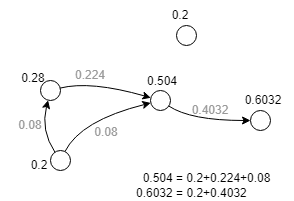## Algorithm Process

### Formula

Using `c` to represent the damping coefficient, the score of node after each round of transfer is: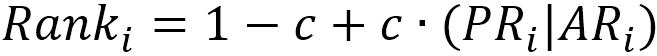Ultipa supports both PageRank and ArticleRank score calculation methods, which can be configured by algorithm parameters.

### Iteration

At the beginning of the algorithm, the initial score of all points is set according to the algorithm parameters; in each iteration, the score is calculated and updated for each node. Iterating and looping by this rule until the score of nodes in the whole graph no longer changes, or the number of iterations reaches the limit.

### Example

Verify the steady state of PageRank calculation with a damping coefficient above, the initial score of nodes in the graph is 1 and damping coefficient is 0.8.

Score Division and Transfer Transfer Result
Initial State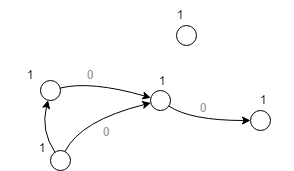1st Iteration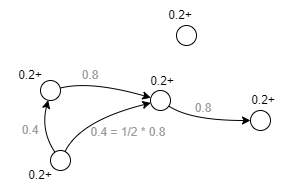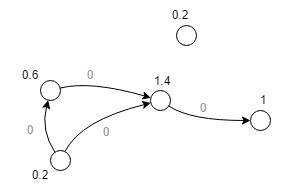2nd Iteration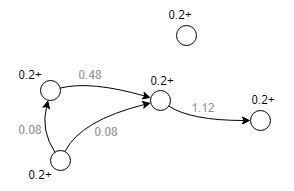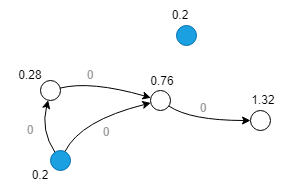3rd Iteration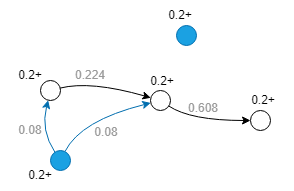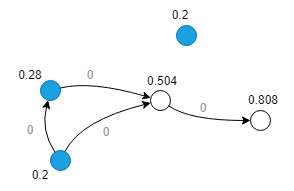4th Iteration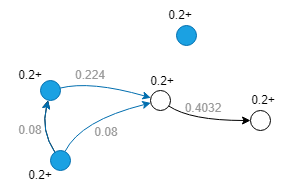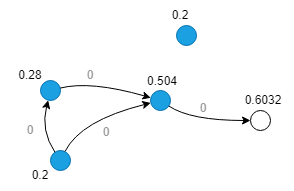5th Iteration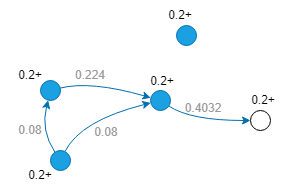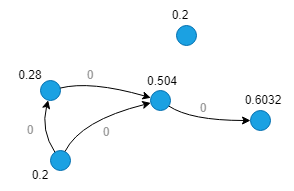## Special Case

### Lonely Node, Disconnected Graph

With the introduction of damping coefficient `c`, the score lonely node gets is always `1-c` (except for the initial state).

There is no score transfer between different connected components in disconnected graph, the score transfer reaches a steady state inside each connected component.

### Self-loop Edge

In PageRank algorithm, each self-loop edge is regarded as a valid outbound degree and a valid inbound degree, that is, self-loop edge of a node passes a part of the score to the node itself, and the execution of this algorithm on the graph with self-loop edge usually requires more iterations to stablize.

### Directed Edge

The score of nodes are divided and passed along the direction of directed edges in PageRank algorithm.

## Command

`algo(page_rank).params(<>)`

Configuration Item Type Default Value Specification Description
init_value float 0.2 >0 Initial score
loop_num int 5 >=1 Number of iterations of the algorithm
damping float 0.8 0~1 Damping coefficient, i.e. the probablity that users continue to stay on the current page for browsing
weaken int 1 1 or 2 1: To calculate PageRank
2: To calculate ArticleRank
limit int -1 -1 or >=0 Number of results to return, -1 means to return all results
order string / ASC or DESC, case insensitive To sort the retuned results, no sorting is applied if not set

Example: Execute PageRank, and set the number of iterations as 3, damping coefficient as 0.7, default score as 1

``````algo(page_rank)
.params({ loop_num: 3, damping: 0.7, init_value: 1, limit: -1 })
``````

## File Writeback

`.write({file: {<>}})`

Parameter Type Default Value Specification Description
filename string / / Name of the file path to be written back. Columns of the file are: `_id``rank`

## Property Writeback

`.write({db: {<>}})`

Parameter Type Default Value Specification Description
property string / / Name of the property to be written back. Write the score back to: `<property>`

## Statistics Writeback

(Not supported)

## Direct Return

`as <alias> return <alias>`

Alias Number Type Description Column Name
0 []perNode Node and its score `_uuid`, `rank`

## Streaming Return

`.stream() as <alias> return <alias>`

Alias Number Type Description Column Name
0 []perNode Node and its score `_uuid`, `rank`

## Real-time Statistics

(Not supported)